## A class XII student appearing for a competitive examination was asked to attempt the following questions. Let a , b  and c  𝑏e three non zero vectors.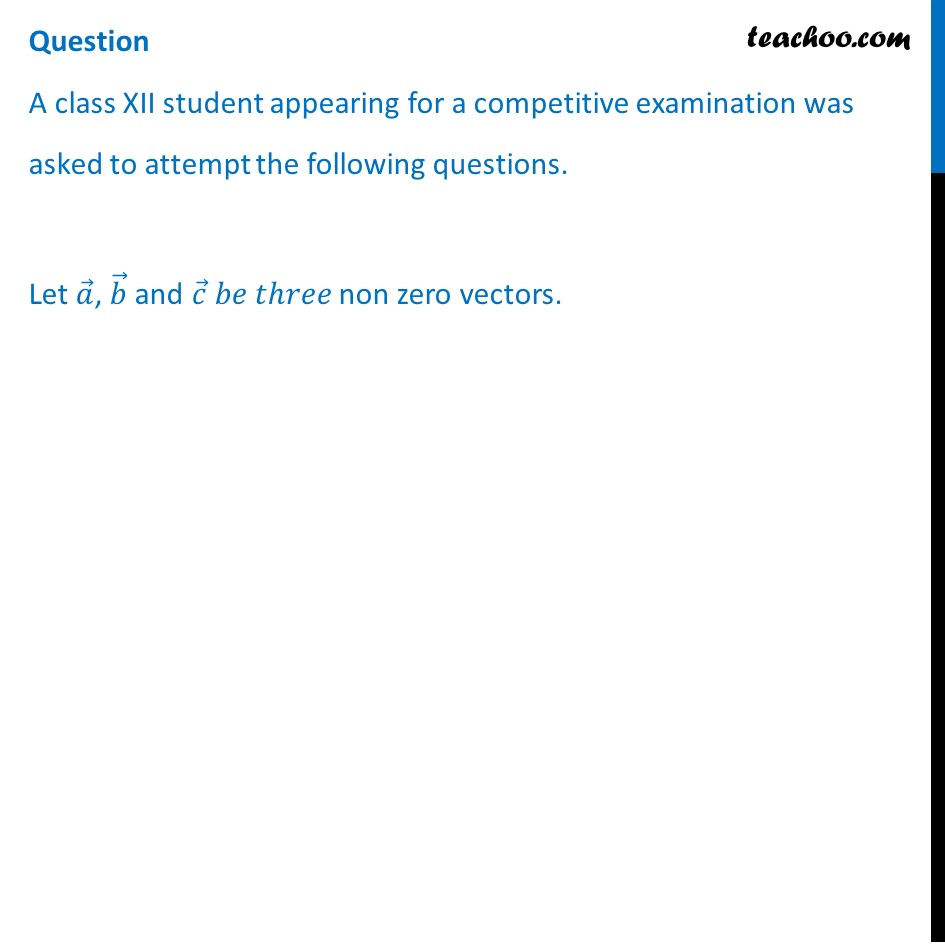## Question 1 If a   and b  are such that|a + b | = |a – b | then (a) a ⊥ b ⃗ (b) a ∥ b ⃗ (c) a = b  (d) None of these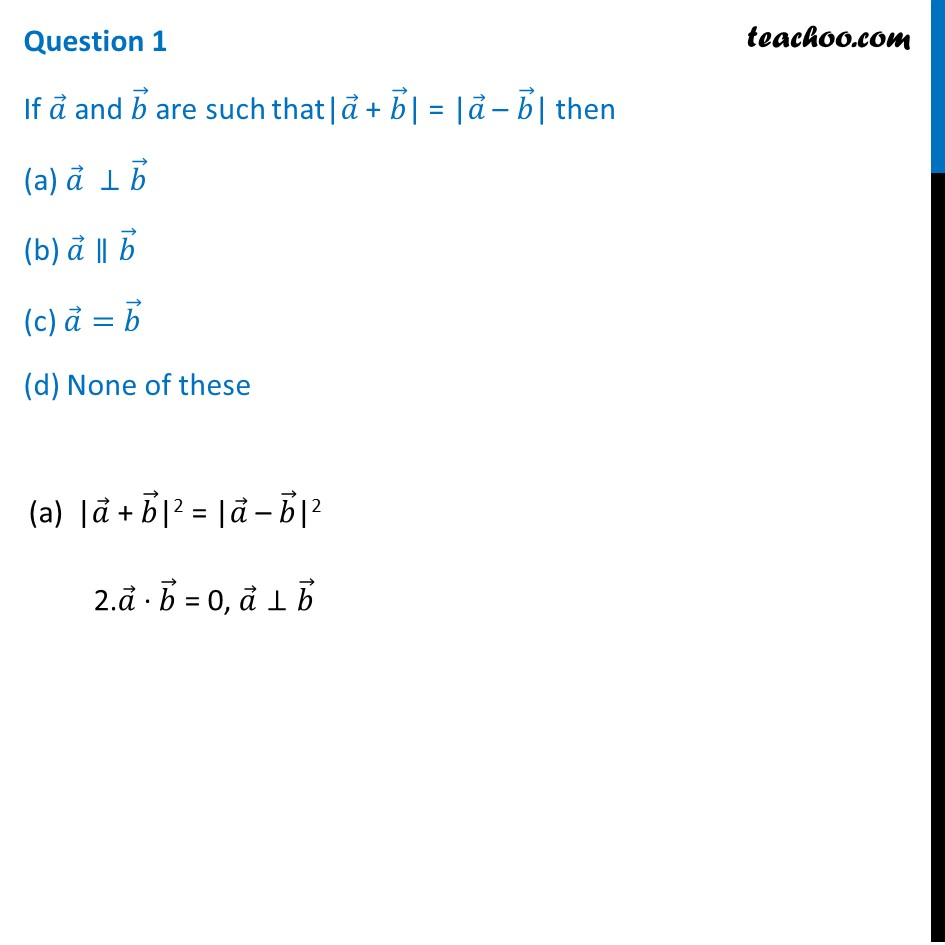## Question 2 If a = i ̂ – 2j ̂, b = 2i ̂ + j ̂ + 3k ̂  then evaluate (2a + b) ∙ [(a + b) × (a − 2b)] (a) 0 (b) 4 (c) 3 (d) 2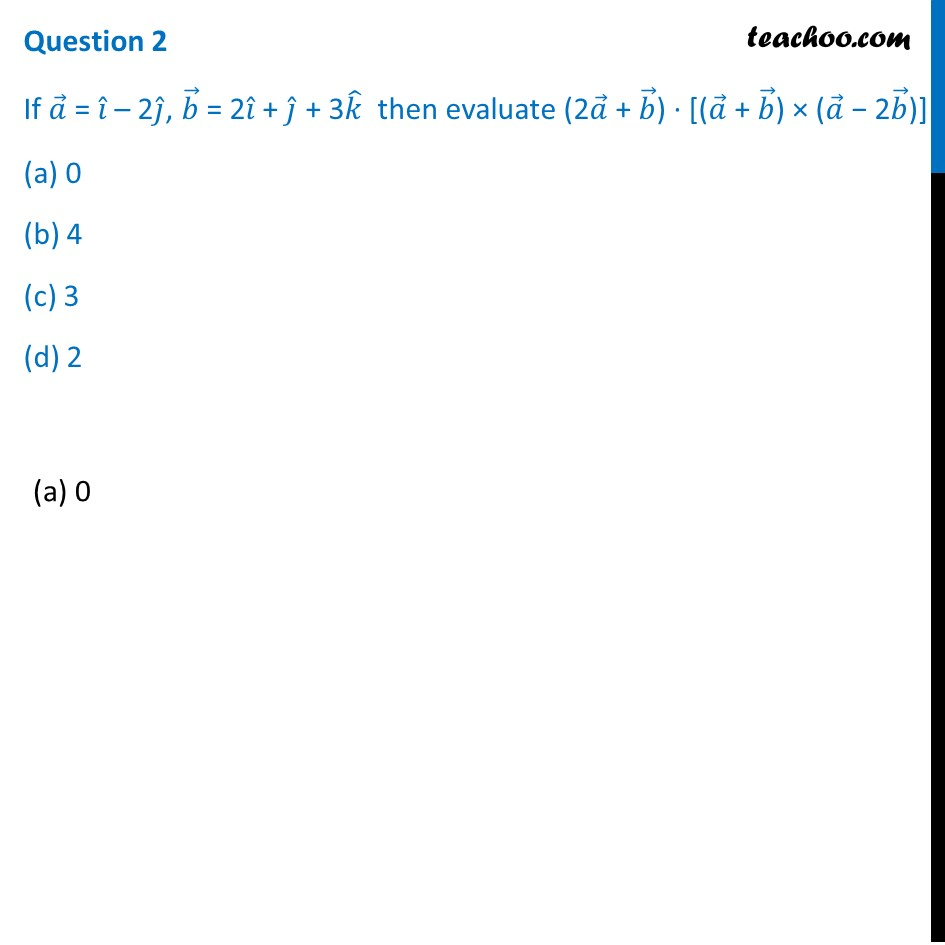## Question 3 If a and b are unit vectors and 𝜃 be the angle between them the, |a ⃗ -b ⃗ | is (a) sin θ/2 (b) 2 sin θ/2 (c) 2 cos θ/2 (d) cos θ/2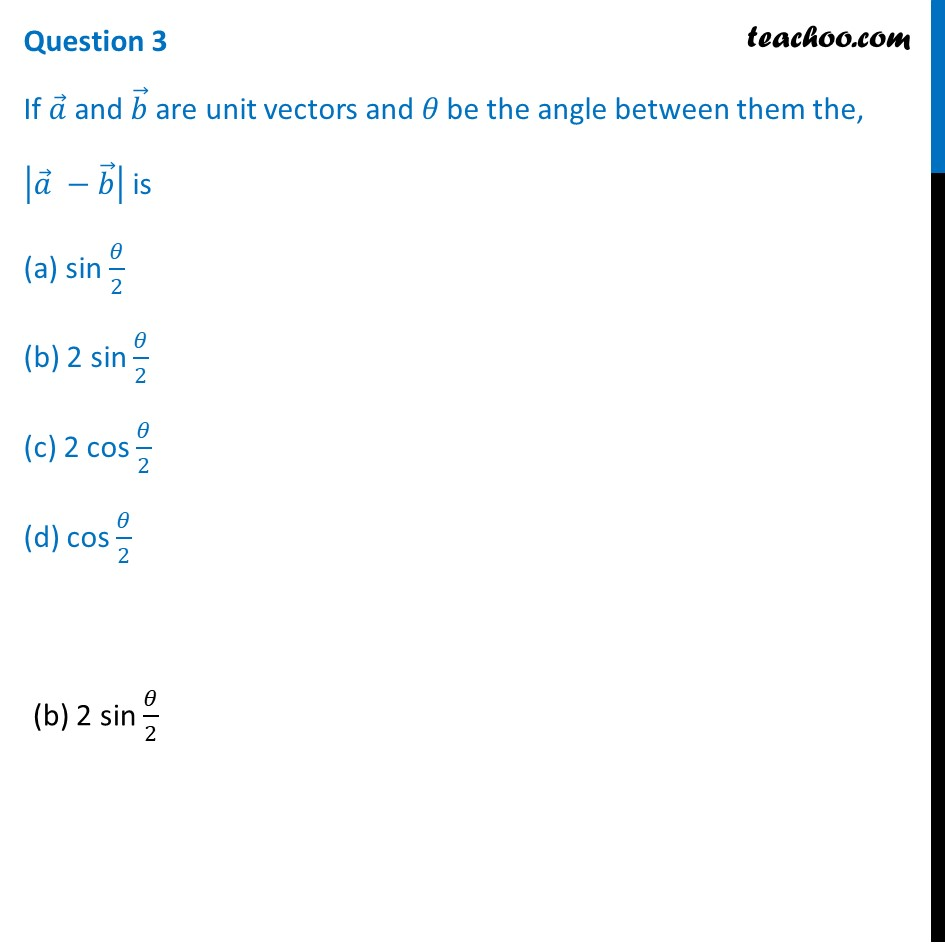## Question 4 Let a, b  and c be unit vectors such that a ∙ b = a ∙ c = 0 and angle between b and c ⃗ is π/6 then a = (a) 2(b × c) (b) –2 (b × c) (c) ±2 (b × c) (d) 2 (b ± c)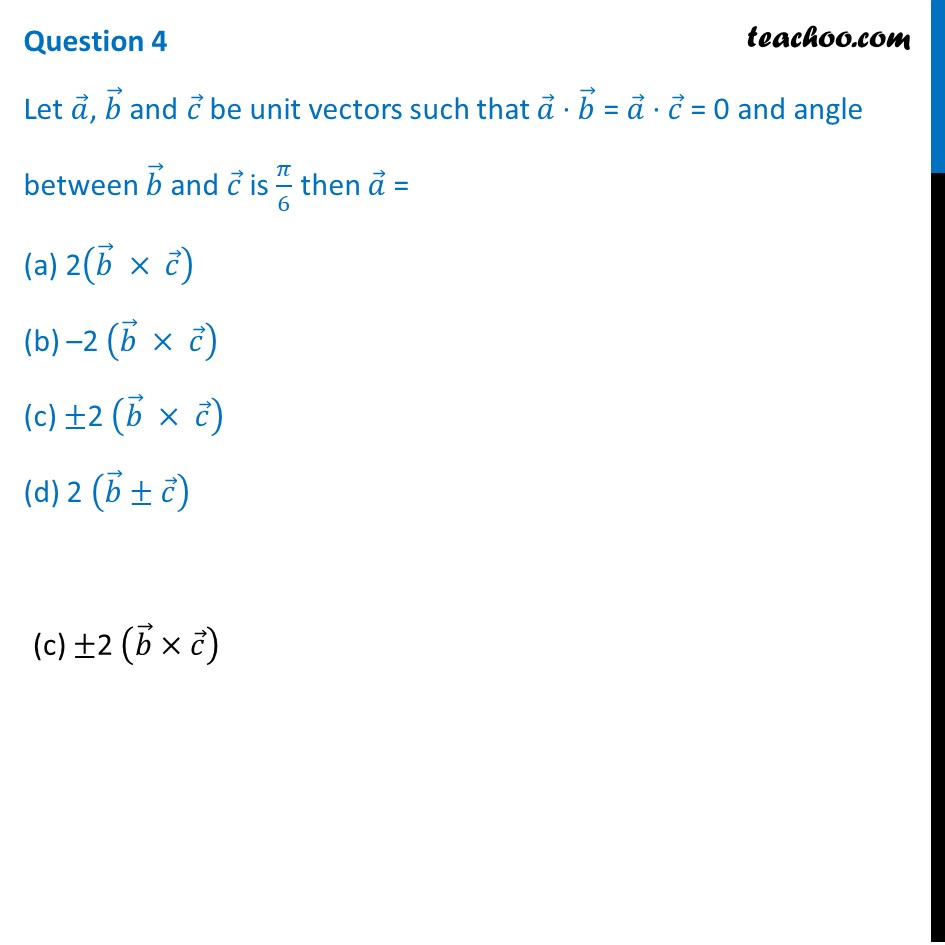## Question 5 The area of the parallelogram formed by a and b as diagonals is (a) 70 (b) 35 (c) √70/2 (d) √70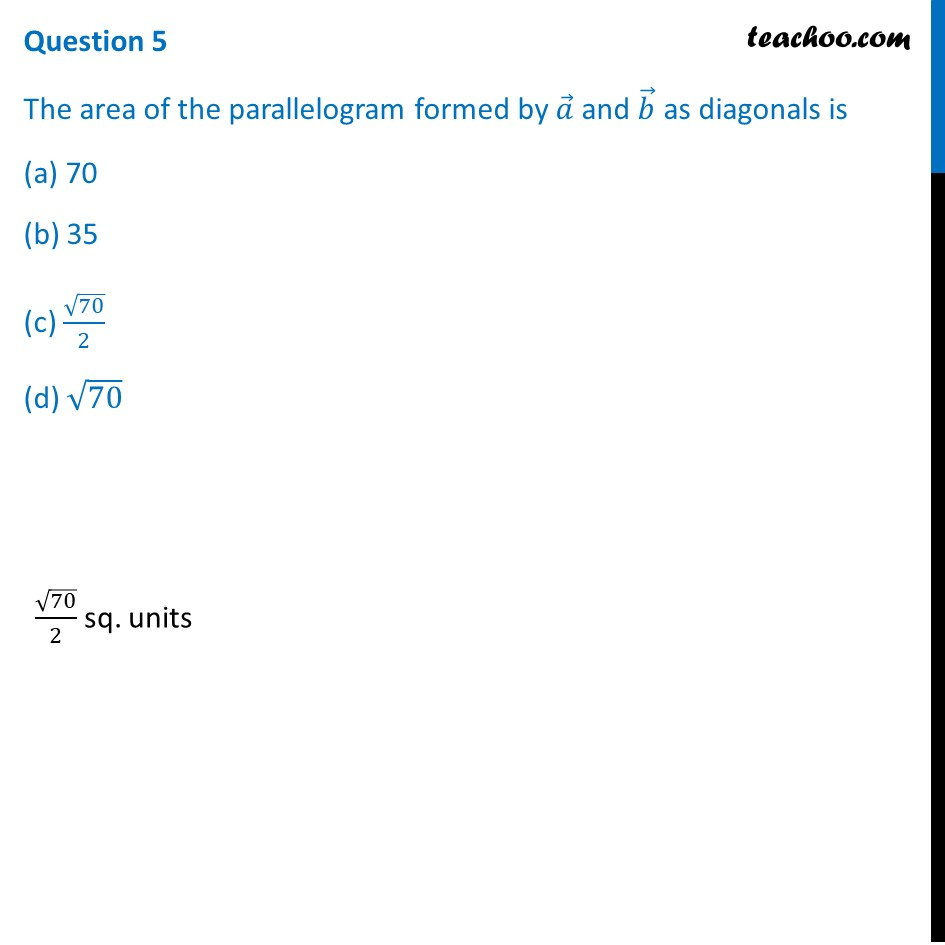1. Chapter 10 Class 12 Vector Algebra (Term 2)
2. Serial order wise
3. Case Based Questions (MCQ)

Transcript

Question A class XII student appearing for a competitive examination was asked to attempt the following questions. Let 𝑎 ⃗, 𝑏 ⃗ and 𝑐 ⃗ 𝑏𝑒 𝑡ℎ𝑟𝑒𝑒 non zero vectors. Question 1 If 𝑎 ⃗ and 𝑏 ⃗ are such that|𝑎 ⃗ + 𝑏 ⃗| = |𝑎 ⃗ – 𝑏 ⃗| then (a) 𝑎 ⃗ ⊥𝑏 ⃗ (b) 𝑎 ⃗∥𝑏 ⃗ (c) 𝑎 ⃗=𝑏 ⃗ (d) None of these|𝑎 ⃗ + 𝑏 ⃗|2 = |𝑎 ⃗ – 𝑏 ⃗|2 2.𝑎 ⃗ ∙ 𝑏 ⃗ = 0, 𝑎 ⃗⊥𝑏 ⃗ Question 2 If 𝑎 ⃗ = 𝑖 ̂ – 2𝑗 ̂, 𝑏 ⃗ = 2𝑖 ̂ + 𝑗 ̂ + 3𝑘 ̂ then evaluate (2𝑎 ⃗ + 𝑏 ⃗) ∙ [(𝑎 ⃗ + 𝑏 ⃗) × (𝑎 ⃗ − 2𝑏 ⃗)] (a) 0 (b) 4 (c) 3 (d) 2(a) 0 Question 3 If 𝑎 ⃗ and 𝑏 ⃗ are unit vectors and 𝜃 be the angle between them the, |𝑎 ⃗ −𝑏 ⃗ | is (a) sin 𝜃/2 (b) 2 sin 𝜃/2 (c) 2 cos 𝜃/2 (d) cos 𝜃/2(b) 2 sin 𝜃/2 Question 4 Let 𝑎 ⃗, 𝑏 ⃗ and 𝑐 ⃗ be unit vectors such that 𝑎 ⃗ ∙ 𝑏 ⃗ = 𝑎 ⃗ ∙ 𝑐 ⃗ = 0 and angle between 𝑏 ⃗ and 𝑐 ⃗ is 𝜋/6 then 𝑎 ⃗ = (a) 2(𝑏 ⃗ × 𝑐 ⃗ ) (b) –2 (𝑏 ⃗ × 𝑐 ⃗ ) (c) ±2 (𝑏 ⃗ × 𝑐 ⃗ ) (d) 2 (𝑏 ⃗±𝑐 ⃗ )(c) ±2 (𝑏 ⃗×𝑐 ⃗ ) Question 5 The area of the parallelogram formed by 𝑎 ⃗ and 𝑏 ⃗ as diagonals is (a) 70 (b) 35 (c) √70/2 (d) √70√70/2 sq. units

Case Based Questions (MCQ)